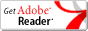Site Designed And Hosted By Digital Data Technologies Inc.
 Home About the Treasurer Tax Search Tax Bill Payment Options Tax Rates Tax Forms Sewer Payments County Home

### Tax Rates

Download Auglaize County Tax Rates for Tax Year 2021 Payable 2022 (PDF)## Tax Calculation

The steps outlined below show how a real estate tax bill is calculated. The calculations used in the example are for a \$100,000 home in Allen Township for tax year 2005; Full tax rates and reduction factors can be found on the tax rate sheet above.

Note: 10% rollback does not apply to commercial property.

Determine the assessed value
Formula: (Appraised Value) x 35% = Assessed Value
Example: \$100,000 x .35 = \$35,000

Divide (Assessed Value) in half to Calculate Half-Year Tax Bill Amount.
Formula: (Appraised Value) / 2 = Assessed Value (For Half-Year Tax Bill Calculation)
Example: \$35,000 / 2 = \$17,500

Calculate the gross tax [See the tax rates page for full rates.]
Formula: (Assessed Value) x (Full Rate) = Gross Tax (for Half-Year) / 1,000
Example: \$17,500 x 83.60 / 1,000 = \$1,463.00

Calculate the reduction factor credit amount [See the tax rates page for reduction factors.]
Formula: (Gross Tax) x (Reduction Factor) = Reduction Factor Credit
Example: \$1,463.00 x .452516 = \$662.03

Reduce the gross tax by the reduction factor credit amount
Formula: (Gross Tax) - (Reduction Factor Credit) = Adjusted Tax
Example: \$1,463.00 - \$662.03 = \$800.97

Calculate the rollback credit amount
Formula: (Adjusted Tax) x (Rollback) = 10% Rollback Reduction
Example: \$800.97 x .10 = \$80.10

For "Owner-Occupied" Residential Properties, there is an additional 2.5% Rollback.
Formula: (Adjusted Tax) x (Rollback) = 2.5% Rollback Reduction
Example: \$800.97 x .025 = \$20.02

Reduce Adjusted Tax by Total Rollback for Half-Year Tax Amount.
Formula: (10% Rollback) + (2.5% Rollback) = Total Rollback Reduction (Adjusted Tax Amount) - (Total Rollback Reduction) = Total Half-Year Tax Amount.
Example: \$80.10 + \$20.02 = \$100.12
\$800.97 - \$100.12 = \$700.85
Half Year Tax Amount: \$700.85 Billed Semi-Annually

 Disclaimer: Records from the Office of the Treasurer are public information under the Ohio Public Records Law. Database last updated: 1/26/2023 10:03:12 PM